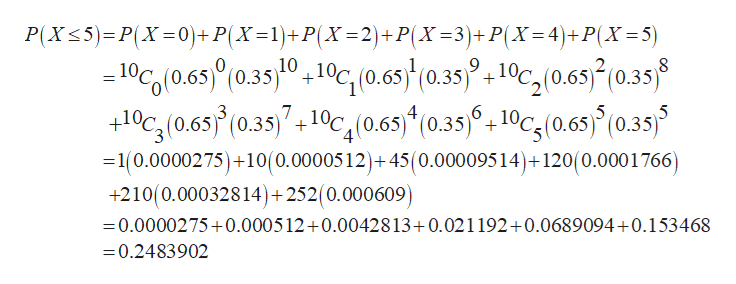# I have the dolling probability problem and have gotten lost .  I can do integers if they are x but not x</= for a binomial probability experiment.please helpN=10, x=c<5, p=.65

Question
26 views

I have the dolling probability problem and have gotten lost .  I can do integers if they are x but not x</= for a binomial probability experiment.

N=10, x=c<5, p=.65

check_circle

Step 1

Consider the given information:

Step 2

Now, apply the formula:

Step 3

Now, solve for provide...help_outlineImage TranscriptioncloseP(X5) P(X0)+P(X-1)+P(X 2)+P(X 3)+P(X=4)+P(X 5) 10C(0.65 /0.3510,10(0.6s0.5102(0.69 (0.35 10C(0.65 (035)10,(0.65 (0.35,10c(0.6 0.35 9 10 + 7 4 5 + =1(0.0000275)+10(0.0000512)+45 (0.00009514)+ 120(0.0001766 +210(0.00032814)+252(0.000609) -0.0000275+0.000512+0.0042813+0.021192+0.0689094+0.153468 =0.2483902 fullscreen

### Want to see the full answer?

See Solution

#### Want to see this answer and more?

Solutions are written by subject experts who are available 24/7. Questions are typically answered within 1 hour.*

See Solution
*Response times may vary by subject and question.
Tagged in

### Probability